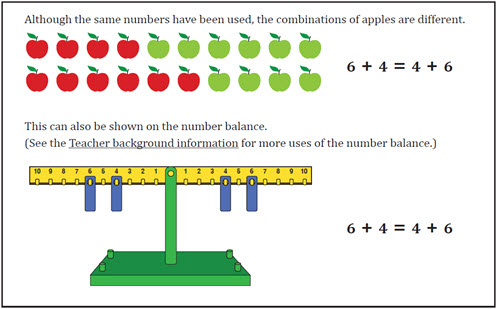Go to website

This sequence of two lessons explores early algebraic thinking around the concept of equivalence. Students develop equivalence understanding by partitioning numbers into two parts. They explore the commutative property and compensation as they are challenged to find all possible combinations. Each lesson is outlined in detail including curriculum links, vocabulary, materials needed, sample answers, discussion points and student resources. This sequence is part of the reSolve: Mathematics by Inquiry program.

Year level(s) Year 1
Audience Teacher
Purpose Teaching resource
Teaching strategies and pedagogical approaches Mathematics investigation
Keywords number patterns, counting, associativity, partitioning

## Curriculum alignment

Curriculum connections Numeracy
Strand and focus Number, Build understanding, Apply understanding
Topics Addition and subtraction, Operating with number
AC: Mathematics (V9.0) content descriptions
AC9M1N03

Quantify sets of objects, to at least 120, by partitioning collections into equal groups using number knowledge and skip counting

AC9M1N04

Add and subtract numbers within 20, using physical and virtual materials, part-part-whole knowledge to 10 and a variety of calculation strategies

Numeracy progression Counting processes (P6)
Number and place value (P3, P4)